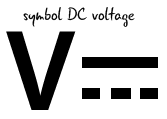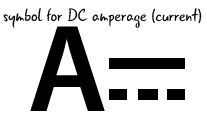## Voltage, Current, Resistance: Review

Let’s look at our water analogy diagram one last time, then quickly review each of the properties we’ve just learned about, what unit it is measured in, and the symbol used to represent it.Name Description Unit Symbol
voltage electromotive force, or the potential flow volts V
current amount of electrical flow amp A
resistance restriction of electrical flow ohm

### How does electricity affect our components?

In a circuit, current, voltage, and resistance are related. If you have current in a system, then there is necessarily a voltage and a resistance. Let’s examine what happens when we reduce only one of these properties.#### Voltage

Remember that voltage represents the potential for electricity to move within a circuit. Voltage will always flow from the highest to lowest charge until it reaches the equilibrium zero state, also known as ground. If we place the same LED and resistor in our circuit, and power it using only 3.3 volts instead of the 5 volts we have been accustomed to, then our LED will be less bright. If we continue to reduce the voltage, our LED will continue to dim until it finally turns off.#### Current

Current is the property related to the flow of electrons in the circuit. Current is what drives our components. What happens if we don’t have enough current within our circuit? Without enough current there are not enough electrons to turn our components on. When you have a flashlight with dead batteries, the batteries have too little current to turn the light on. If we reduce the current to our circuit by adding resistors, the LED will turn off suddently once the minimum current needed to turn the LED on disappears.#### Resistance

Resistance is a measure of how a material restricts the flow of electricity. All materials naturally have some resistance, but if the resistance is too high the electrical flow will be stopped altogether. However, if there is too little resistance our components can be overwhelmed by the amount of current and fry. We often use resistors to restrict the flow in order to preserve the other components of our circuits. If we add more resistors or change the value of the resistors in the basic circuit with the LED, we will increase resistance value and decrease the amount of electricity that reaches our LED, perhaps even limiting our LED’s ability to produce any light.

Let’s look quickly at how some of components we are familiar with relate to voltage, current and resistance.

### How are our components affected by a change in electrical properties?

Let’s take a quick look at how our components are affected by changes in electrical properties.

Component Image Voltage Current Resistance
LEDThe LED will get dimmer as the voltage gets lower, or brighter as more voltage is added; if there is too much voltage the LED will burn out LEDs only need a very small amount of current to run. However, reducing the amount of current too much will turn the LED off. LEDs have a tiny amount of resistance
ResistorVoltage is converted into heat when it crosses over a resistor. More voltage means more heat and less voltage means less heat. Resistors lower the amount of current being drawn in a circuit, The amount of resistance depends on the resistor’s rated value. Check appendix b to learn how to identify resistor values
BatteryBatteries establish the voltage level for both the high point and zero volts, a.k.a. the ground. Current comes from the battery. The current flowing will change depending on what components attached to the battery and how much current they require. Since a battery is not a perfect conductor, there is a small amount of resistance inside of the battery, but when it is in our circuits it is effectively zero.

Now let’s take a look at how voltage, current and resistance interact with each other in a rule called Ohm’s Law.